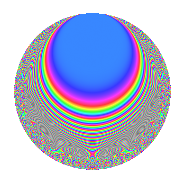# Properties

 Label 23.3.dLevel 23 Weight 3 Character orbit d Rep. character $$\chi_{23}(5,\cdot)$$ Character field $$\Q(\zeta_{22})$$ Dimension 30 Newform subspaces 1 Sturm bound 6 Trace bound 0

# Related objects

## Defining parameters

 Level: $$N$$ = $$23$$ Weight: $$k$$ = $$3$$ Character orbit: $$[\chi]$$ = 23.d (of order $$22$$ and degree $$10$$) Character conductor: $$\operatorname{cond}(\chi)$$ = $$23$$ Character field: $$\Q(\zeta_{22})$$ Newform subspaces: $$1$$ Sturm bound: $$6$$ Trace bound: $$0$$

## Dimensions

The following table gives the dimensions of various subspaces of $$M_{3}(23, [\chi])$$.

Total New Old
Modular forms 50 50 0
Cusp forms 30 30 0
Eisenstein series 20 20 0

## Trace form

 $$30q - 11q^{2} - 11q^{3} - 23q^{4} - 11q^{5} + 22q^{6} - 11q^{7} + 10q^{8} - 38q^{9} + O(q^{10})$$ $$30q - 11q^{2} - 11q^{3} - 23q^{4} - 11q^{5} + 22q^{6} - 11q^{7} + 10q^{8} - 38q^{9} - 11q^{10} - 11q^{11} - 14q^{12} - 11q^{13} - 11q^{14} + 66q^{15} + 73q^{16} + 44q^{17} + 126q^{18} + 22q^{19} + 77q^{20} + 22q^{21} + 36q^{23} - 22q^{24} - 152q^{25} - 186q^{26} - 62q^{27} - 275q^{28} - 88q^{29} - 363q^{30} - 110q^{31} - 147q^{32} - 132q^{33} + 231q^{34} + 209q^{35} + 229q^{36} + 341q^{37} + 374q^{38} + 295q^{39} + 429q^{40} + 77q^{41} + 319q^{42} + 77q^{43} + 110q^{44} - 99q^{46} - 110q^{47} - 550q^{48} - 422q^{49} - 396q^{50} - 275q^{51} - 472q^{52} - 187q^{53} - 198q^{54} - 165q^{55} + 176q^{56} - 176q^{57} - 13q^{58} - q^{59} + 539q^{60} + 297q^{61} + 82q^{62} + 264q^{63} + 386q^{64} + 220q^{65} + 264q^{66} + 11q^{67} - 66q^{69} - 198q^{70} - 176q^{71} - 605q^{72} - 121q^{73} - 352q^{74} + 154q^{75} + 110q^{76} + 110q^{77} + 360q^{78} + 33q^{79} - 242q^{80} + 494q^{81} + 96q^{82} - 154q^{83} + 11q^{84} + 275q^{85} + 143q^{86} + 271q^{87} + 429q^{88} + 121q^{89} + 242q^{90} + 166q^{92} + 260q^{93} - 295q^{94} - 154q^{95} - 419q^{96} + 154q^{97} + 77q^{98} - 242q^{99} + O(q^{100})$$

## Decomposition of $$S_{3}^{\mathrm{new}}(23, [\chi])$$ into newform subspaces

Label Dim. $$A$$ Field CM Traces $q$-expansion
$$a_2$$ $$a_3$$ $$a_5$$ $$a_7$$
23.3.d.a $$30$$ $$0.627$$ None $$-11$$ $$-11$$ $$-11$$ $$-11$$

## Hecke Characteristic Polynomials

There are no characteristic polynomials of Hecke operators in the database